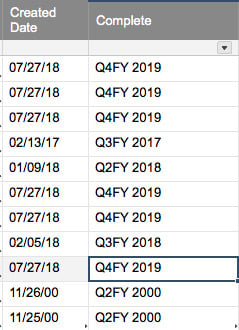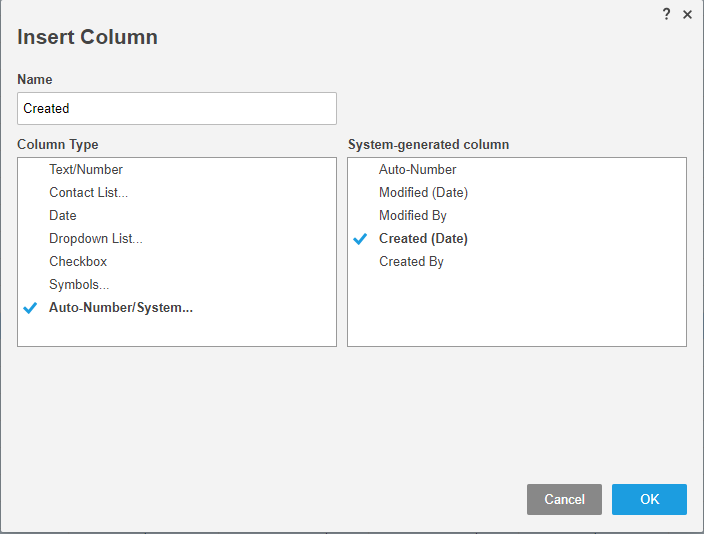# return fiscal quarter from auto generated date column

Hi, this formula returns a blank - what is wrong?

=IF(OR(MONTH([Created Date]9) = 8, MONTH([Created Date]9) = 9, MONTH([Created Date]9) = 10), "Q1FY " + YEAR([Created Date]9), IF(OR(MONTH([Created Date]9) = 11, MONTH([Created Date]9) = 12, MONTH([Created Date]9) = 1), "Q2FY " + YEAR([Created Date]9), IF(OR(MONTH([Created Date]9) = 2, MONTH([Created Date]9) = 3, MONTH([Created Date]9) = 4), "Q3FY " + YEAR([Created Date]9), IF(OR(MONTH([Created Date]9) = 5, MONTH([Created Date]9) = 6, MONTH([Created Date]9) = 7), "Q4FY " + (VALUE(YEAR([Created Date]9) + 1))))))

the auto generated date in the Created Date column is 7/27/18

Tags:

• Hi Lisa. It seems to work for me. I get Q4FY 2019. I'm not sure why you're getting a blank. How is the date being auto-generated?• from a form... would that matter? and btw it has the time as well.

• It shouldn't matter. I can't think of any reason why it would be returning a blank...

• Whenever you are specifically referencing the date portion in a Created (date) type column, you need to use a DATEONLY function. Something along the lines of...

=IF(OR(MONTH(DATEONLY([Created Date]9)) = 8, MONTH(DATEONLY([Created Date]9)) = 9, .........................................

The auto generated date in the Created (date) type column in your case is not being generated by the form itself, but by the addition of another row to the sheet. If you were to skip the form and manually enter data directly into a new row on the sheet, it would still auto generate a date/time stamp of when that row was created.

• Thanks!

but now getting Incorrect Argument for:

=IF(AND([Created Date]7 >= DATEONLY(2018, 8, 1), [Created Date]7 <= DATEONLY(2018, 10, 31)), "FY18Q1",

IF(AND([Created Date]7 >= DATEONLY(2018, 11, 1), [Created Date]7 <= DATEONLY(2019, 1, 31)), "FY18Q2",

IF(AND([Created Date]7 >= DATEONLY(2019, 2, 1), [Created Date]7 <= DATEONLY(2019, 4, 30)), "FY18Q3",

IF(AND([Created Date]7 >= DATEONLY(2019, 5, 1), [Created Date]7 <= DATEONLY(2019, 7, 31)), "FY18Q4"))))

any ideas?

• You have the DATEONLY in the wrong spot. Try switching it to...

DATEONLY([Created Date]@row) >= DATE(2019, 2, 1)

• getting unparseable now...

=IF(AND DATEONLY([Created Date]7) >= DATE(2018, 8, 1), DATEONLY([Created Date]7) <= DATE(2018, 10, 31)), "FY18Q1",

IF(AND DATEONLY(([Created Date]7) >= DATE(2018, 11, 1), DATEONLY([Created Date]7) <= DATE(2019, 1, 31)), "FY18Q2",

IF(AND DATEONLY(([Created Date]7) >= DATE(2019, 2, 1), DATEONLY([Created Date]7) <= DATE (2019, 4, 30)), "FY18Q3",

IF(AND DATEONLY(([Created Date]7) >= DATE(2019, 5, 1), DATEONLY([Created Date]7) <= DATE(2019, 7, 31)), "FY18Q4"))))

can't tell you how much I appreciate you guys trying to help me.  thanks, Lisa

• You're missing the ( beween AND and DATEONLY.

................AND(DATEONLY(..............

=IF(AND(DATEONLY([Created Date]7) >= DATE(2018, 8, 1), DATEONLY([Created Date]7) <= DATE(2018, 10, 31)), "FY18Q1",

IF(AND(DATEONLY([Created Date]7) >= DATE(2018, 11, 1), DATEONLY([Created Date]7) <= DATE(2019, 1, 31)), "FY18Q2",

IF(AND(DATEONLY([Created Date]7) >= DATE(2019, 2, 1), DATEONLY([Created Date]7) <= DATE (2019, 4, 30)), "FY18Q3",

IF(AND(DATEONLY([Created Date]7) >= DATE(2019, 5, 1), DATEONLY([Created Date]7) <= DATE(2019, 7, 31)), "FY18Q4"))))

• unparseable...

=IF(AND(DATEONLY([Created Date]7) >= DATE(2018, 8, 1), DATEONLY([Created Date]7) <= DATE(2018, 10, 31)), "FY18Q1",IF(AND(DATEONLY([Created Date]7) >= DATE(2018, 11, 1), DATEONLY([Created Date]7) <= DATE(2019, 1, 31)), "FY18Q2",IF(AND(DATEONLY([Created Date]7) >= DATE(2019, 2, 1), DATEONLY([Created Date]7) <= DATE (2019, 4, 30)), "FY18Q3",IF(AND(DATEONLY([Created Date]7) >= DATE(2019, 5, 1), DATEONLY([Created Date]7) <= DATE(2019, 7, 31)), "FY18Q4"))))

• Your Created Date column is an Auto-number/System column (see screenshot below), correct?

What is the ACTUAL column name? You should be using that in place of [Created Date].

Those are the only two things that I can think of that could be causing the problem. Everything else looks good to go...• It is the System-Generated date and it is actually named Created Date.  I'm at a loss.  Could it be a parenthesis or space somewhere?

=IF(AND(DATEONLY([Created Date]7)) >= DATE(2018, 8, 1), DATEONLY([Created Date]7) <= DATE(2018, 10, 31), "FY18Q1",

IF(AND(DATEONLY([Created Date]7) >= DATE(2018, 11, 1), DATEONLY([Created Date]7) <= DATE(2019, 1, 31)), "FY18Q2",

IF(AND(DATEONLY([Created Date]7) >= DATE(2019, 2, 1), DATEONLY([Created Date]7) <= DATE (2019, 4, 30)), "FY18Q3",

IF(AND(DATEONLY([Created Date]7) >= DATE(2019, 5, 1), DATEONLY([Created Date]7) <= DATE(2019, 7, 31)), "FY18Q4")))))

• In your most recent comment, your closing AND parenthesis is in the wrong position. I am also not sure if you are copy/pasting from here or not, but in some of the formulas going back and forth here, it is broken down into multiple lines using the "Enter" key instead of only appearing to be multiple lines because of the length. Try below.

=IF(AND(DATEONLY([Created Date]7) >= DATE(2018, 8, 1), DATEONLY([Created Date]7) <= DATE(2018, 10, 31)), "FY18Q1", IF(AND(DATEONLY([Created Date]7) >= DATE(2018, 11, 1), DATEONLY([Created Date]7) <= DATE(2019, 1, 31)), "FY18Q2", IF(AND(DATEONLY([Created Date]7) >= DATE(2019, 2, 1), DATEONLY([Created Date]7) <= DATE (2019, 4, 30)), "FY18Q3", IF(AND(DATEONLY([Created Date]7) >= DATE(2019, 5, 1), DATEONLY([Created Date]7) <= DATE(2019, 7, 31)), "FY18Q4"))))

• I found a rogue extra space, deleted it and now no error!

THANK YOU!!!

• Excellent! Glad you got it figured out. Happy to help.## Help Article Resources

Want to practice working with formulas directly in Smartsheet?

Check out the Formula Handbook template!